# CS 3343 Analysis of Algorithms Lecture 19 Introduction

• Slides: 38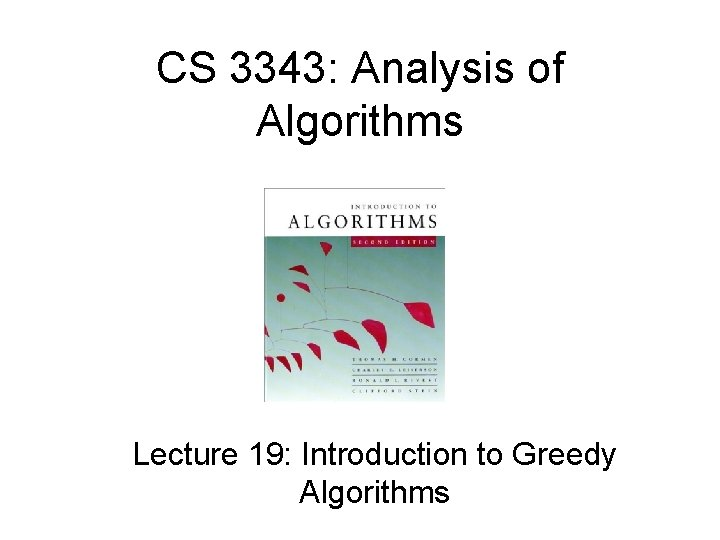CS 3343: Analysis of Algorithms Lecture 19: Introduction to Greedy Algorithms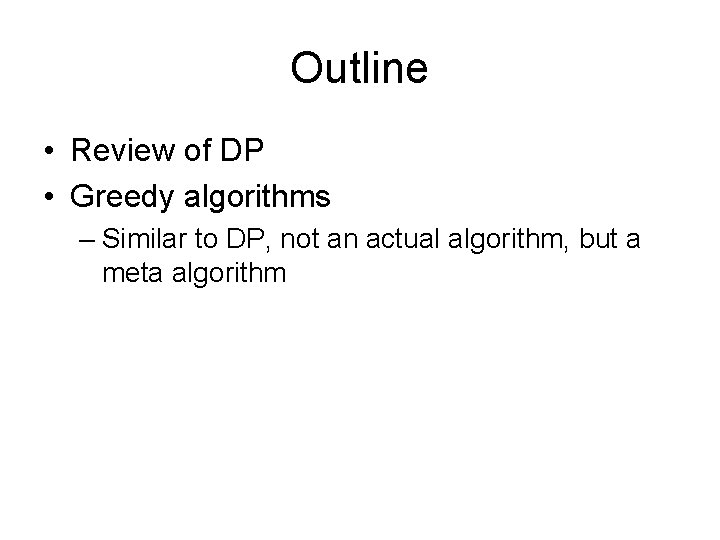Outline • Review of DP • Greedy algorithms – Similar to DP, not an actual algorithm, but a meta algorithmTwo steps to dynamic programming • Formulate the solution as a recurrence relation of solutions to subproblems. • Specify an order of evaluation for the recurrence so you always have what you need.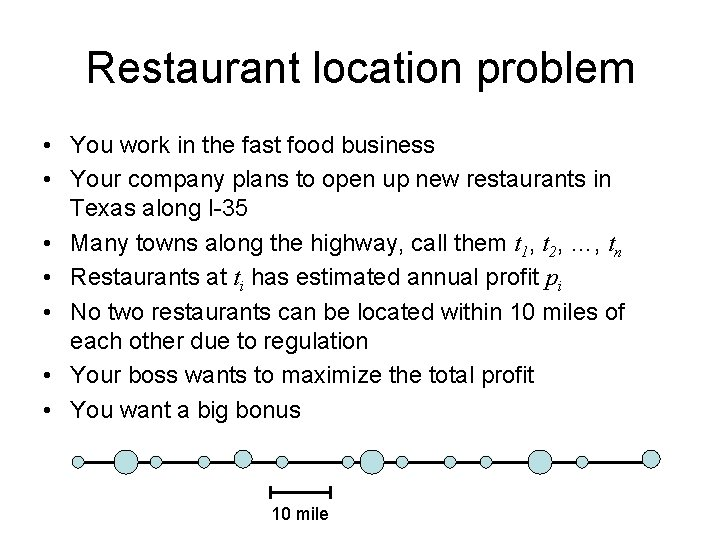Restaurant location problem • You work in the fast food business • Your company plans to open up new restaurants in Texas along I-35 • Many towns along the highway, call them t 1, t 2, …, tn • Restaurants at ti has estimated annual profit pi • No two restaurants can be located within 10 miles of each other due to regulation • Your boss wants to maximize the total profit • You want a big bonus 10 mileA DP algorithm • Suppose you’ve already found the optimal solution • It will either include tn or not include tn • Case 1: tn not included in optimal solution – Best solution same as best solution for t 1 , …, tn-1 • Case 2: tn included in optimal solution – Best solution is pn + best solution for t 1 , …, tj , where j < n is the largest index so that dist(tj, tn) ≥ 10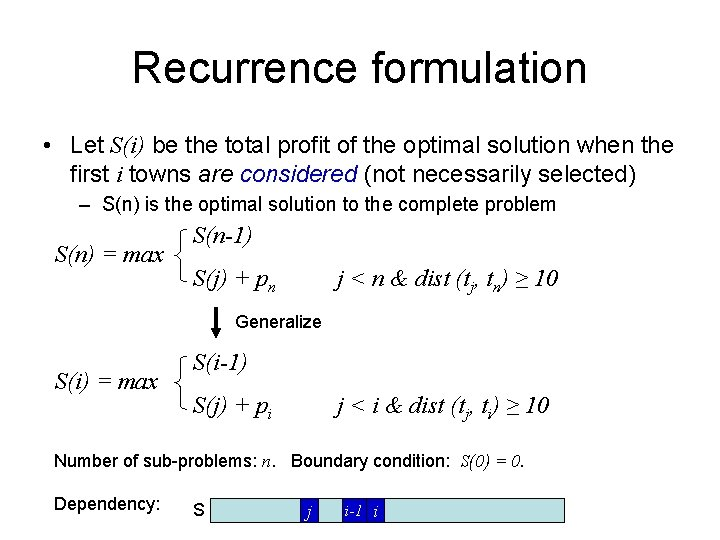Recurrence formulation • Let S(i) be the total profit of the optimal solution when the first i towns are considered (not necessarily selected) – S(n) is the optimal solution to the complete problem S(n) = max S(n-1) S(j) + pn j < n & dist (tj, tn) ≥ 10 Generalize S(i) = max S(i-1) S(j) + pi j < i & dist (tj, ti) ≥ 10 Number of sub-problems: n. Boundary condition: S(0) = 0. Dependency: S j i-1 iExample Distance (mi) dummy 100 0 Profit (100 k) S(i) 5 2 2 6 7 6 3 6 7 9 8 3 6 7 9 9 10 10 7 4 3 12 2 4 12 5 12 12 14 26 26 Optimal: 26 S(i) = max S(i-1) S(j) + pi j < i & dist (tj, ti) ≥ 10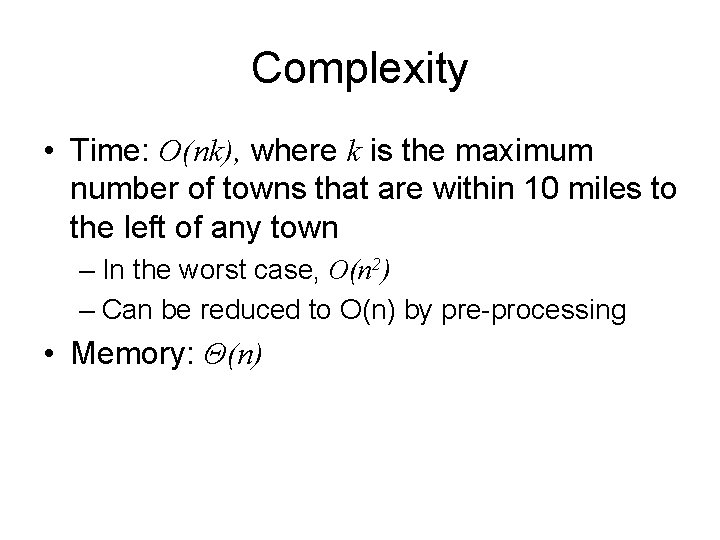Complexity • Time: O(nk), where k is the maximum number of towns that are within 10 miles to the left of any town – In the worst case, O(n 2) – Can be reduced to O(n) by pre-processing • Memory: Θ(n)Knapsack problem • Each item has a value and a weight • Objective: maximize value • Constraint: knapsack has a weight limitation Three versions: 0 -1 knapsack problem: take each item or leave it Fractional knapsack problem: items are divisible Unbounded knapsack problem: unlimited supplies of each item. Which one is easiest to solve? We studied the 0 -1 problem.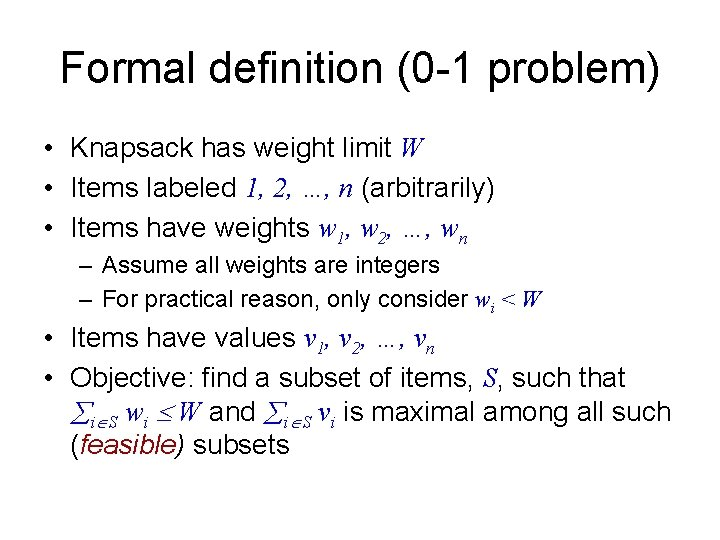Formal definition (0 -1 problem) • Knapsack has weight limit W • Items labeled 1, 2, …, n (arbitrarily) • Items have weights w 1, w 2, …, wn – Assume all weights are integers – For practical reason, only consider wi < W • Items have values v 1, v 2, …, vn • Objective: find a subset of items, S, such that i S wi W and i S vi is maximal among all such (feasible) subsets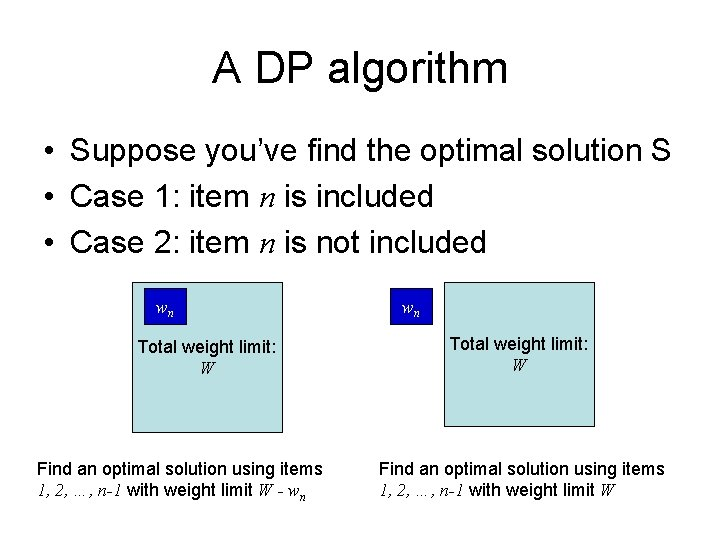A DP algorithm • Suppose you’ve find the optimal solution S • Case 1: item n is included • Case 2: item n is not included wn Total weight limit: W Find an optimal solution using items 1, 2, …, n-1 with weight limit W - wn wn Total weight limit: W Find an optimal solution using items 1, 2, …, n-1 with weight limit WRecursive formulation • Let V[i, w] be the optimal total value when items 1, 2, …, i are considered for a knapsack with weight limit w => V[n, W] is the optimal solution V[n, W] = max V[n-1, W-wn] + vn V[n-1, W] Generalize V[i, w] = max V[i-1, w-wi] + vi item i is taken V[i-1, w] item i not taken V[i-1, w] if wi > w item i not taken Boundary condition: V[i, 0] = 0, V[0, w] = 0. Number of sub-problems = ?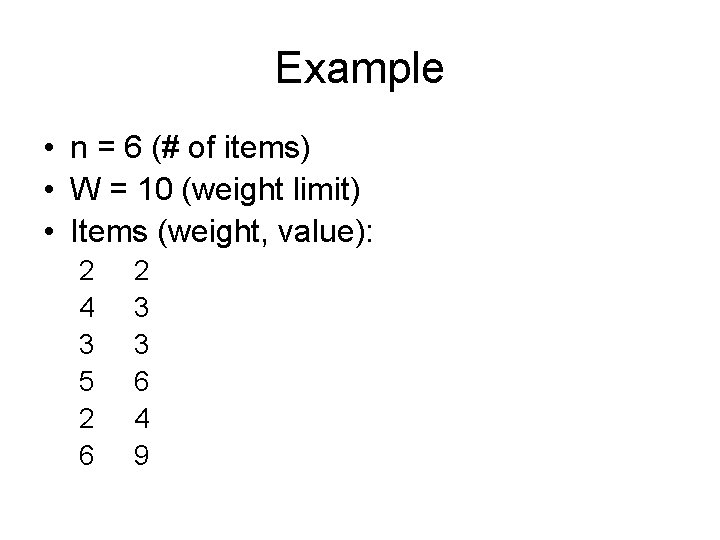Example • n = 6 (# of items) • W = 10 (weight limit) • Items (weight, value): 2 4 3 5 2 6 2 3 3 6 4 9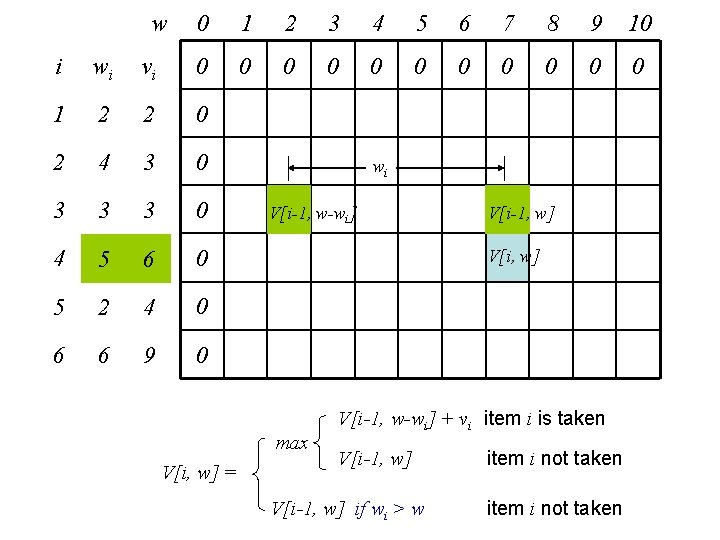w 0 1 2 3 4 5 6 7 8 9 10 0 0 i wi vi 0 1 2 2 0 2 4 3 0 3 3 3 0 4 5 6 0 5 2 4 0 6 6 9 0 wi V[i-1, w-wi] V[i, w] max V[i, w] = V[i-1, w] V[i-1, w-wi] + vi item i is taken V[i-1, w] if wi > w item i not taken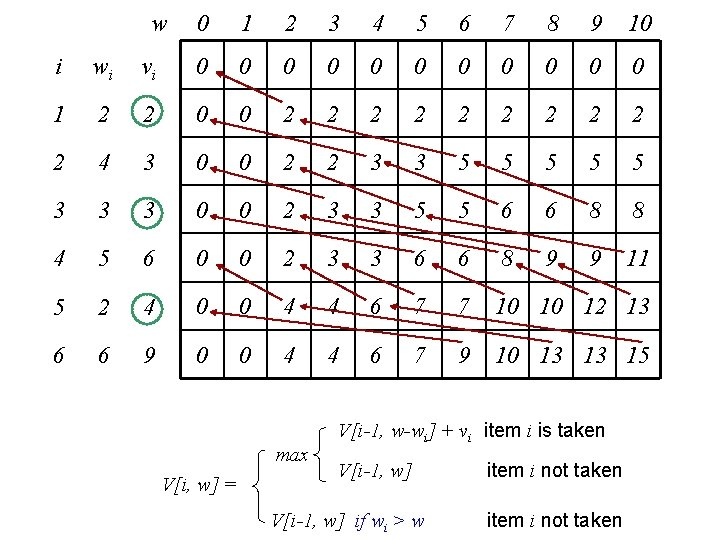w 0 1 2 3 4 5 6 7 8 9 10 i wi vi 0 0 0 1 2 2 0 0 2 2 2 2 2 4 3 0 0 2 2 3 3 5 5 5 3 3 3 0 0 2 3 3 5 5 6 6 8 8 4 5 6 0 0 2 3 3 6 6 8 9 9 11 5 2 4 0 0 4 4 6 7 7 10 10 12 13 6 6 9 0 0 4 4 6 7 9 10 13 13 15 max V[i, w] = V[i-1, w-wi] + vi item i is taken V[i-1, w] if wi > w item i not taken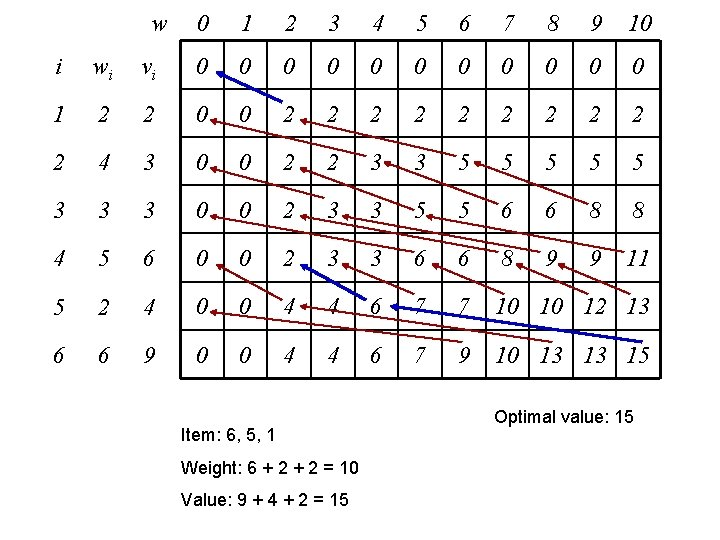w 0 1 2 3 4 5 6 7 8 9 10 i wi vi 0 0 0 1 2 2 0 0 2 2 2 2 2 4 3 0 0 2 2 3 3 5 5 5 3 3 3 0 0 2 3 3 5 5 6 6 8 8 4 5 6 0 0 2 3 3 6 6 8 9 9 11 5 2 4 0 0 4 4 6 7 7 10 10 12 13 6 6 9 0 0 4 4 6 7 9 10 13 13 15 Item: 6, 5, 1 Weight: 6 + 2 = 10 Value: 9 + 4 + 2 = 15 Optimal value: 15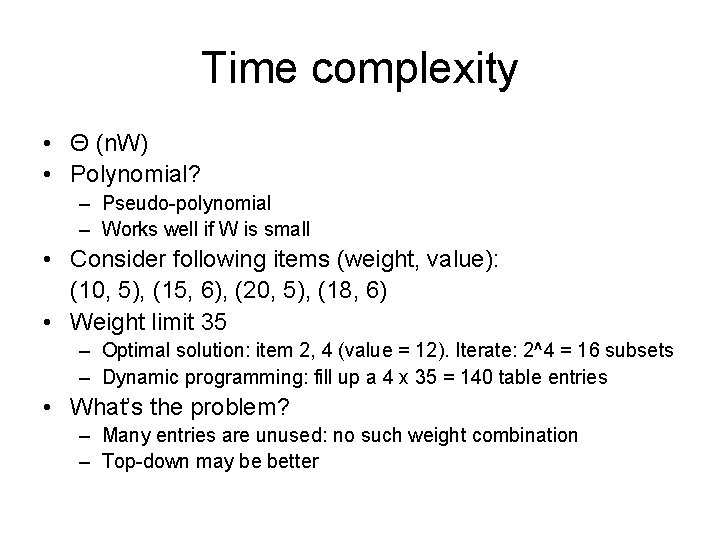Time complexity • Θ (n. W) • Polynomial? – Pseudo-polynomial – Works well if W is small • Consider following items (weight, value): (10, 5), (15, 6), (20, 5), (18, 6) • Weight limit 35 – Optimal solution: item 2, 4 (value = 12). Iterate: 2^4 = 16 subsets – Dynamic programming: fill up a 4 x 35 = 140 table entries • What’s the problem? – Many entries are unused: no such weight combination – Top-down may be better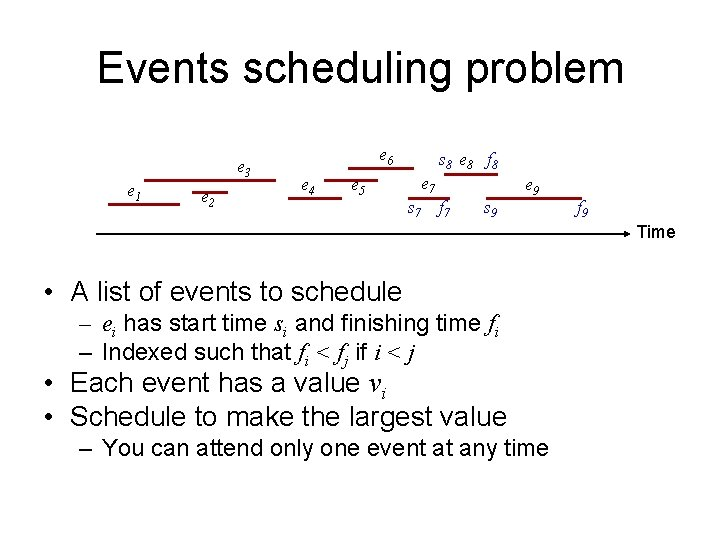Events scheduling problem e 3 e 1 e 2 e 6 e 4 e 5 s 8 e 8 f 8 e 7 s 7 f 7 s 9 e 9 f 9 Time • A list of events to schedule – ei has start time si and finishing time fi – Indexed such that fi < fj if i < j • Each event has a value vi • Schedule to make the largest value – You can attend only one event at any time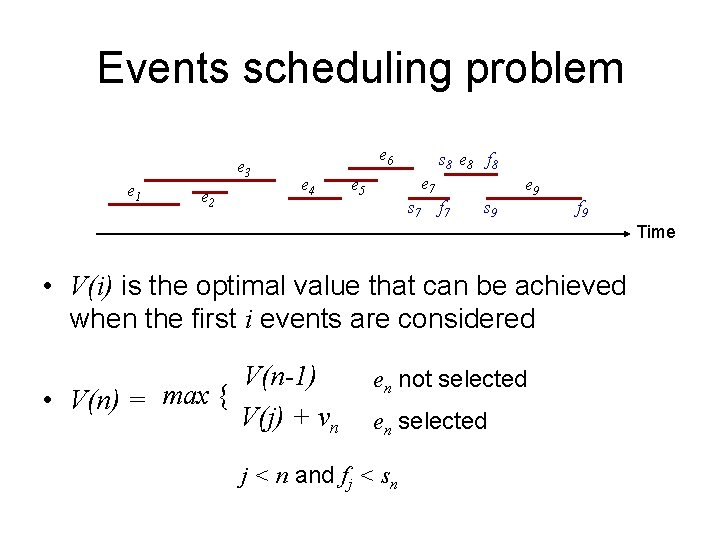Events scheduling problem e 3 e 1 e 2 e 6 e 4 s 8 e 8 f 8 e 7 e 5 s 7 f 7 s 9 e 9 f 9 Time • V(i) is the optimal value that can be achieved when the first i events are considered V(n-1) • V(n) = max { V(j) + vn en not selected en selected j < n and fj < sn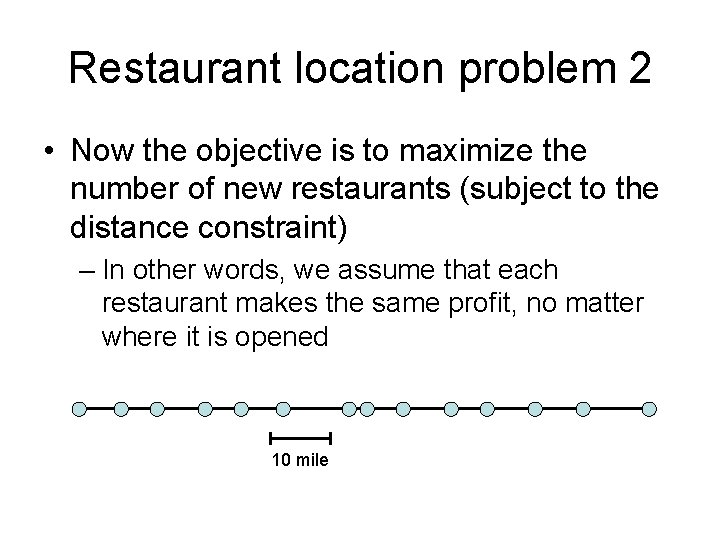Restaurant location problem 2 • Now the objective is to maximize the number of new restaurants (subject to the distance constraint) – In other words, we assume that each restaurant makes the same profit, no matter where it is opened 10 mileA DP Algorithm • Exactly as before, but pi = 1 for all i S(i) = max S(i-1) S(j) + pi j < i & dist (tj, ti) ≥ 10 S(i-1) S(j) + 1 j < i & dist (tj, ti) ≥ 10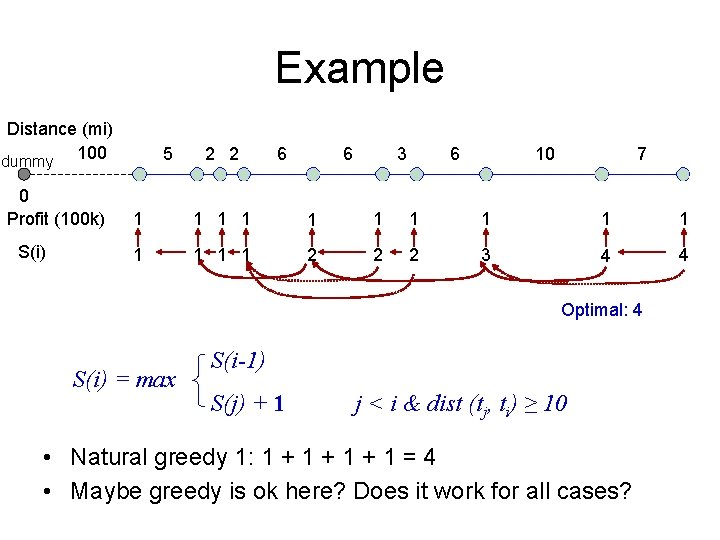Example Distance (mi) dummy 100 0 Profit (100 k) S(i) 5 2 2 6 6 3 6 10 7 1 1 1 1 2 2 2 3 4 4 Optimal: 4 S(i) = max S(i-1) S(j) + 1 j < i & dist (tj, ti) ≥ 10 • Natural greedy 1: 1 + 1 + 1 = 4 • Maybe greedy is ok here? Does it work for all cases?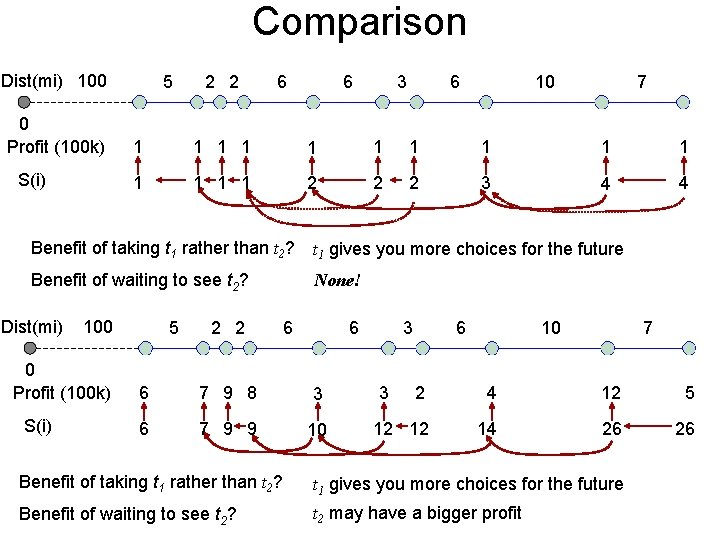Comparison Dist(mi) 100 0 Profit (100 k) S(i) 5 2 2 6 6 3 6 10 7 1 1 1 1 2 2 2 3 4 4 Benefit of taking t 1 rather than t 2? t 1 gives you more choices for the future Benefit of waiting to see t 2? None! Dist(mi) 100 0 Profit (100 k) S(i) 5 2 2 6 6 6 7 9 8 3 6 7 9 9 10 3 3 6 10 7 2 4 12 5 12 12 14 26 26 Benefit of taking t 1 rather than t 2? t 1 gives you more choices for the future Benefit of waiting to see t 2? t 2 may have a bigger profit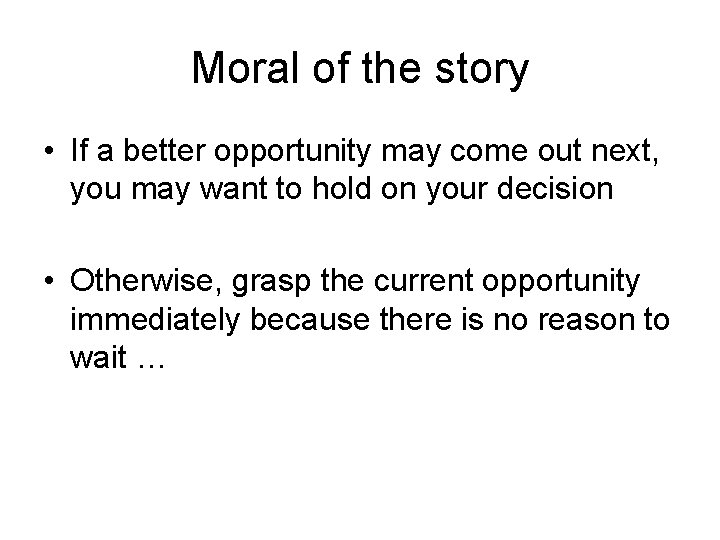Moral of the story • If a better opportunity may come out next, you may want to hold on your decision • Otherwise, grasp the current opportunity immediately because there is no reason to wait …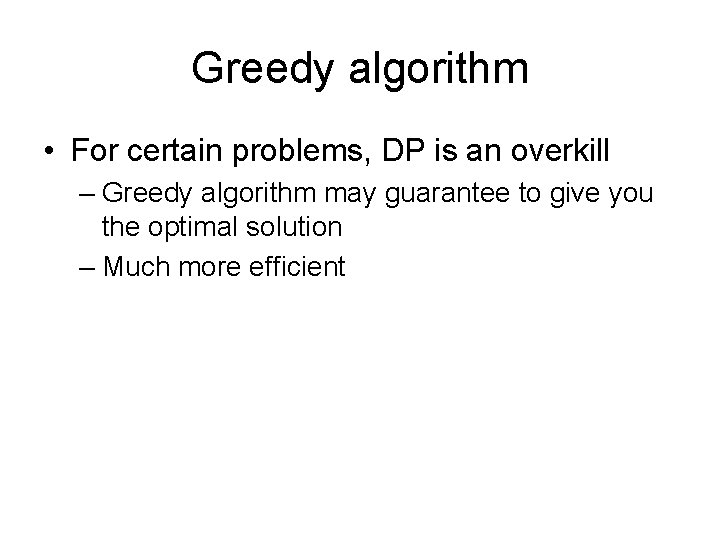Greedy algorithm • For certain problems, DP is an overkill – Greedy algorithm may guarantee to give you the optimal solution – Much more efficient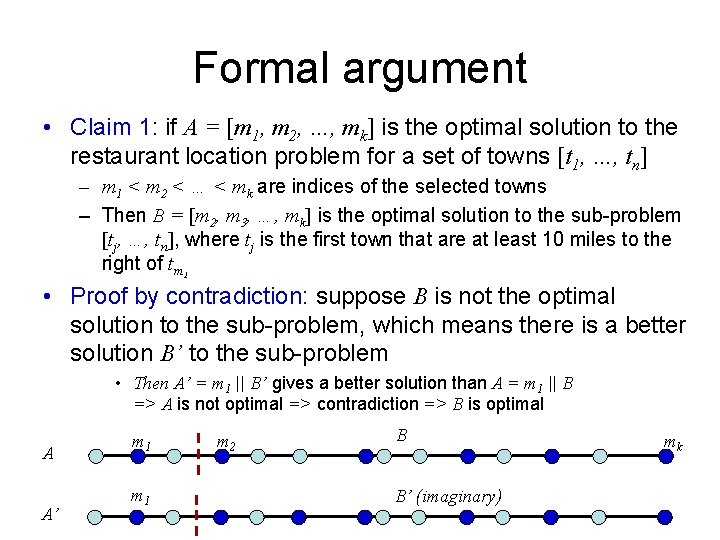Formal argument • Claim 1: if A = [m 1, m 2, …, mk] is the optimal solution to the restaurant location problem for a set of towns [t 1, …, tn] – m 1 < m 2 < … < mk are indices of the selected towns – Then B = [m 2, m 3, …, mk] is the optimal solution to the sub-problem [tj, …, tn], where tj is the first town that are at least 10 miles to the right of tm 1 • Proof by contradiction: suppose B is not the optimal solution to the sub-problem, which means there is a better solution B’ to the sub-problem • Then A’ = m 1 || B’ gives a better solution than A = m 1 || B => A is not optimal => contradiction => B is optimal A A’ m 1 m 2 B B’ (imaginary) mkImplication of Claim 1 • If we know the first town that needs to be chosen, we can reduce the problem to a smaller sub-problem – This is similar to dynamic programming – Optimal substructureFormal argument (cont’d) • Claim 2: for the uniform-profit restaurant location problem, there is an optimal solution that chooses t 1 • Proof by contradiction: suppose that no optimal solution can be obtained by choosing t 1 – Say the first town chosen by the optimal solution S is ti, i > 1 – Replace ti with t 1 will not violate the distance constraint, and the total profit remains the same => S’ is an optimal solution – Contradiction – Therefore claim 2 is valid S S’Implication of Claim 2 • We can simply choose the first town as part of the optimal solution – This is different from DP – Decisions are made immediately • By Claim 1, we then only need to repeat this strategy to the remaining sub-problem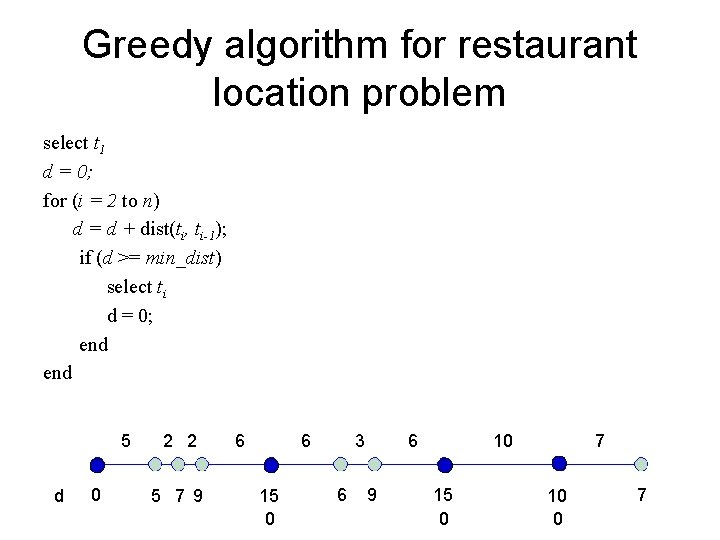Greedy algorithm for restaurant location problem select t 1 d = 0; for (i = 2 to n) d = d + dist(ti, ti-1); if (d >= min_dist) select ti d = 0; end 5 d 0 2 2 5 7 9 6 6 15 0 3 6 6 9 10 15 0 7 10 0 7Complexity • Time: Θ(n) • Memory: – Θ(n) to store the input – Θ(1) for greedy selection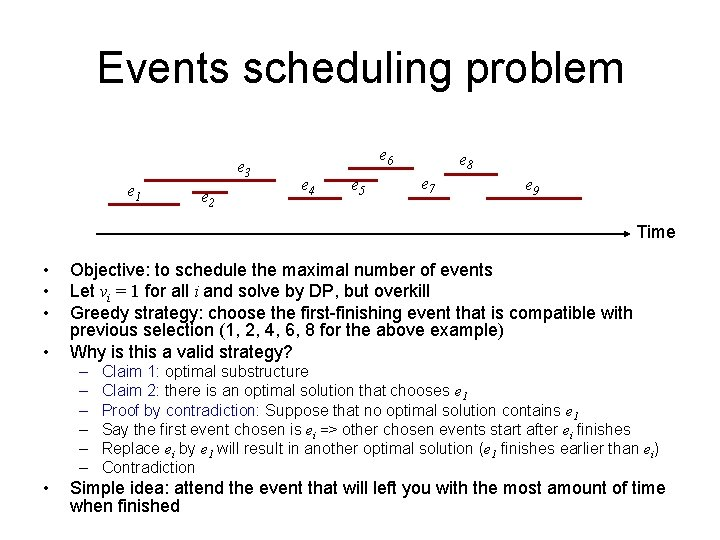Events scheduling problem e 3 e 1 e 2 e 6 e 4 e 5 e 8 e 7 e 9 Time • • Objective: to schedule the maximal number of events Let vi = 1 for all i and solve by DP, but overkill Greedy strategy: choose the first-finishing event that is compatible with previous selection (1, 2, 4, 6, 8 for the above example) Why is this a valid strategy? – – – • Claim 1: optimal substructure Claim 2: there is an optimal solution that chooses e 1 Proof by contradiction: Suppose that no optimal solution contains e 1 Say the first event chosen is ei => other chosen events start after ei finishes Replace ei by e 1 will result in another optimal solution (e 1 finishes earlier than ei) Contradiction Simple idea: attend the event that will left you with the most amount of time when finished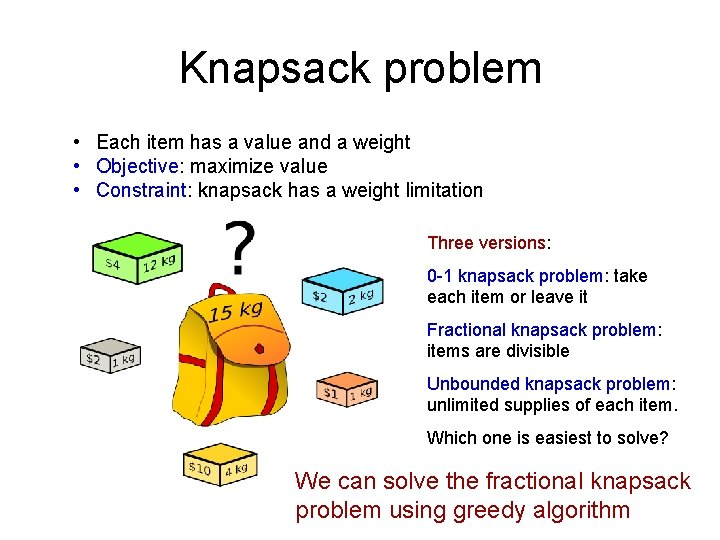Knapsack problem • Each item has a value and a weight • Objective: maximize value • Constraint: knapsack has a weight limitation Three versions: 0 -1 knapsack problem: take each item or leave it Fractional knapsack problem: items are divisible Unbounded knapsack problem: unlimited supplies of each item. Which one is easiest to solve? We can solve the fractional knapsack problem using greedy algorithmGreedy algorithm for fractional knapsack problem • Compute value/weight ratio for each item • Sort items by their value/weight ratio into decreasing order – Call the remaining item with the highest ratio the most valuable item (MVI) • Iteratively: – If the weight limit can not be reached by adding MVI • Select MVI – Otherwise select MVI partially until weight limit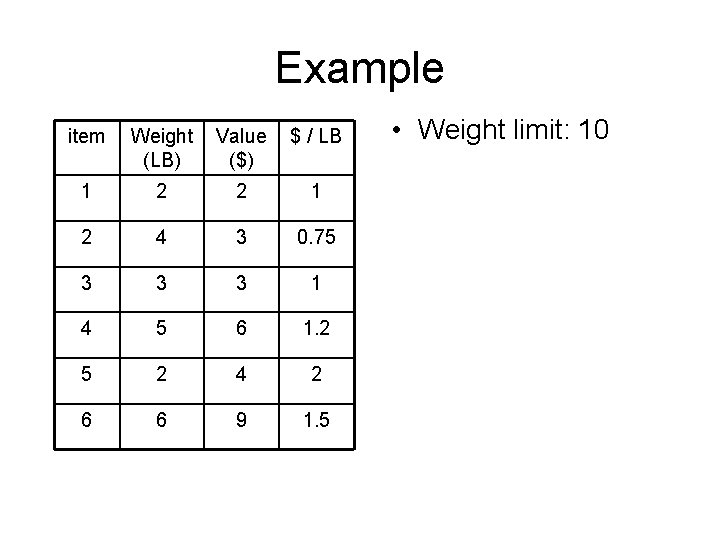Example item Weight (LB) Value (\$) \$ / LB 1 2 2 1 2 4 3 0. 75 3 3 3 1 4 5 6 1. 2 5 2 4 2 6 6 9 1. 5 • Weight limit: 10Example • Weight limit: 10 item Weight (LB) Value (\$) \$ / LB 5 2 4 2 6 6 9 1. 5 – 2 LB, \$4 4 5 6 1. 2 • Take item 6 1 2 2 1 3 3 3 1 2 4 3 0. 75 • Take item 5 – 8 LB, \$13 • Take 2 LB of item 4 – 10 LB, 15. 4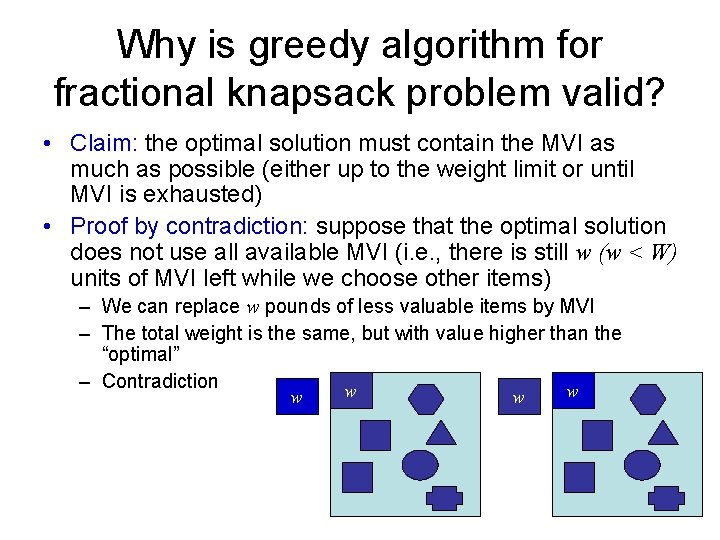Why is greedy algorithm for fractional knapsack problem valid? • Claim: the optimal solution must contain the MVI as much as possible (either up to the weight limit or until MVI is exhausted) • Proof by contradiction: suppose that the optimal solution does not use all available MVI (i. e. , there is still w (w < W) units of MVI left while we choose other items) – We can replace w pounds of less valuable items by MVI – The total weight is the same, but with value higher than the “optimal” – Contradiction w wElements of greedy algorithm 1. Optimal substructure 2. Locally optimal decision leads to globally optimal solution • For most optimization problems, greedy algorithm will not guarantee an optimal solution • But may give you a good starting point to use other optimization techniques • Starting from next week, we’ll study several problems in graph theory that can actually be solved by greedy algorithm